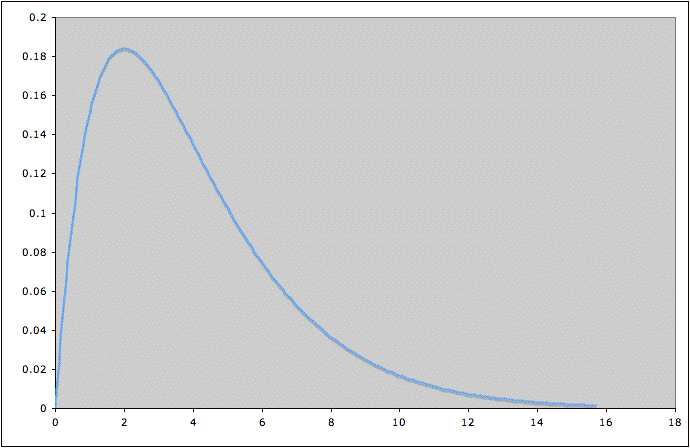COMMON MISTEAKS MISTAKES IN USING STATISTICS: Spotting and Avoiding Them

# Random Variables and Probability Distributions

I. In most applications, a random variable can be thought of as a variable that depends on a random process. Here are some examples to help explain the concepts involved:

1. Toss a die and look at what number is on the side that lands up.
Tossing the die is an example of a random process; the number on top is the random variable.

2. Toss two dice and take the sum of the numbers that land up.
Tossing the dice is the random process; the sum is the random variable.

3. Toss two dice and take the product of the numbers that land up.
Tossing the dice is the random process; the product is the random variable.

Examples 2 and 3 together show that the same random process can be involved in two different random variables.

4. Randomly pick (in a way that gives each student an equal chance of being chosen) a UT student and measure their height.
Picking the student is the random process; their height is the random variable.

5. Randomly pick (in a way that gives each student an equal chance of being chosen) a student in a particular class and measure their height.
Picking the student is the random process; their height is the random variable.

Examples 4 and 5 illustrate that using the same variable (in this case, height) but different random processes (in this case, choosing from different populations) gives different random variables.

6. Measure the height of the third student who walks into this class.
In all the examples before this one, the random process was done deliberately; in Example 6, the random process is one that occurs naturally.1

Because Examples 5 and 6 involved different random processes, they are different random variables.

7. Toss a coin and see whether it comes up heads or tails.
Tossing the coin is the random process; the variable is heads or tails.

Example 7 shows that a random variable doesn't necessarily have to take on numerical values.

II. Usually, some values of a random variable occur more frequently than others. For example, if we are talking about heights of university students, heights of around 5' 7" are much more common that heights of around 4' or heights around 7'. In other words, some values of the random variable occur with higher probability than others. This can be represented graphically by the probability distribution of the random variable. For example, a random variable might have a probability distribution that looks like this:The possible values for the random variable are along the horizontal axis. The height of the curve above a possible value roughly tells how likely the nearby values are. This particular distribution tells us that values of the random variable around 2 (where the curve is highest) are most common, and that very large values (where the curve is lowest) are uncommon. More precisely, the area under the curve between two values a and b is the probability that the random variable will take on values between a and b. In this example, we can see that the value of the random variable is much more likely to lie between 2 and 4 (where the curve is high) than between 12 and 14 (where the curve is low).

Notes:
1. See footnote 1 on the page What Is a Random Sample?